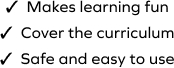# Subtract the Subtraction Sentences

SplashLearn - The complete PreK - Grade 5 Math & ELA Learning Program Built for Your Child
Home > Subtract the Subtraction Sentences
The game challenges young mathematicians to hone their math skills by solving a set of problems on subtraction to find answers to a group of problems. It's important that students work at a level appropriate for them. This game requires learners to work with numbers within 20. Students will drag and drop the items at the correct places to solve the problems.